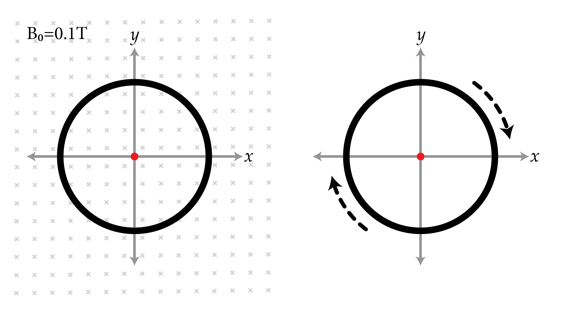# This is how I hula hoop

A uniformly charged nonconducting loop is placed so its center is at the origin. The normal to the loop is $\hat{z}$. There is also a homogeneous magnetic field of strength $B_{0}=0.1~\mbox{T}$ in the -$\hat{z}$ direction (i.e. perpendicular to the plane of the loop). This is shown in the figure on the left. Suddenly, the magnetic field is switched off and the loop begins to rotate about the z-axis as in the figure on the right. Determine the magnitude of the loop's final angular speed in rad/s if the specific charge to mass ratio of the loop is $q/m= 10^{-1}~\mbox{C/kg}$.×